# Difference between revisions of "Curvature of direct sum of connections equals direct sum of curvatures"

Suppose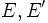$E,E'$ are vector bundles over a differential manifold$M$. Suppose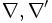$\nabla,\nabla'$ are connections on$E,E'$ respectively. Let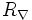$R_\nabla$ denote the Riemann curvature tensor of$\nabla$ and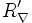$R_\nabla'$ denote that Riemann curvature tensor of$\nabla'$. Then if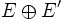$E \oplus E'$ is the direct sum of vector bundles,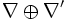$\nabla \oplus \nabla'$ is the direct sum of connections, and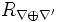$R_{\nabla \oplus \nabla'}$ is its Riemann curvature tensor, we have: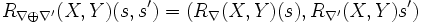$R_{\nabla \oplus \nabla'}(X,Y)(s ,s') = \left(R_\nabla(X,Y)(s),R_{\nabla'}(X,Y)s'\right)$.